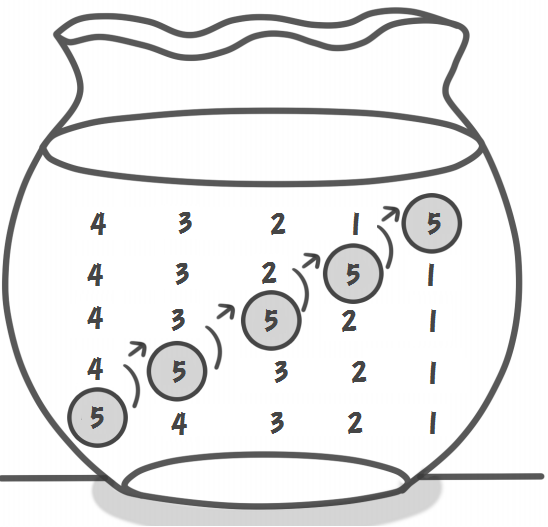# [通俗易懂的C++基础算法] 冒泡排序

## 冒泡排序

### 基本思想

aaaaaaz! 不愧是度娘，能把这么简单的一个算法写这么长的介绍，下面我简单说一下：

### 举个栗子

1: [5  4] 3  2  1   比较5与4，因为5<4不正确，所以交换
2:  4 [5  3] 2  1   比较5与3，因为5<3不正确，所以交换
3:  4  3 [5  2] 1   比较5与2，因为5<2不正确，所以交换
4:  4  3  2 [5  1]  比较5与1，因为5<1不正确，所以交换
4  3  2  1  5   第一轮的结果### 交换

#### 中介变量

void Swap(int & a,int & b) {
int t=a;
a=b;
b=a;
}


#### 加减

void Swap(int & a,int & b) {
a=a+b; // a=x+y b=y
b=a-b; // a=x+y b=x+y-y=x
a=a-b; // a=x+y-x=y b=x;
}

void Swap(int & a,int & b) {
a=a-b; // a=x-y b=y
b=a+b; // a=x-y b=(x-y)+y=x
a=b-a; // a=x-(x-y)=y b=x;
}


### 模板代码

const int maxn=1e5; // 数组大小
int a[maxn]; // 数组a 从下标1 开始存数字

void swap(int & a,int & b) { int t=a;a=b,b=t; }

void BubbleSort(int n) { // 排序 在数组 a 中从 1-n 的数字
bool Sorted=false; // 排序完成的标志
while(!Sorted) {
//
for(int i=2;i<=n;i++) {
if(a[i-1]>a[i]) {
swap(a[i-1],a[i]);
}
}
// 判断是否排序完成
Sorted=true; // 默认当成排序完成了，如果检查的时候发现没完成再把标志变为未完成
for(int i=2;i<=n;i++) {
if(a[i-1]>a[i]) {
Sorted=false;
}
}
}
return ;
}


### 题目

Luogu P1116 车厢重组

const int maxn=1e4+11; // 防止溢出 习惯性写上比题目范围大一点的数字就行
int a[maxn];

int BubbleSort(int n) // 返回交换的次数
int count=0; // 计数器，每次交换都加一
bool Sorted=false;
while(!Sorted) {
for(int i=2;i<=n;i++) {
if(a[i-1]>a[i]) {
swap(a[i-1],a[i]);
count+=1; // 计数
}
}
Sorted=true;
for(int i=2;i<=n;i++) {
if(a[i-1]>a[i]) {
Sorted=false;
}
}
}
return count;
}

10-30444
11-181292
06-0523
01-06105
03-0796
06-0681
07-08712
04-141250
07-04275
06-1286
07-04861
07-1255
08-02213
11-04469
08-121423
12-10328
09-29295
09-2688

### “相关推荐”对你有帮助么？

•非常没帮助
•没帮助
•一般
•有帮助
•非常有帮助

©️2022 CSDN 皮肤主题：游动-白 设计师：我叫白小胖被折叠的  条评论 为什么被折叠?到【灌水乐园】发言CornWorld

¥2 ¥4 ¥6 ¥10 ¥20余额支付 (余额：-- )扫码支付获取中扫码支付点击重新获取扫码支付1.余额是钱包充值的虚拟货币，按照1:1的比例进行支付金额的抵扣。
2.余额无法直接购买下载，可以购买VIP、C币套餐、付费专栏及课程。余额充值